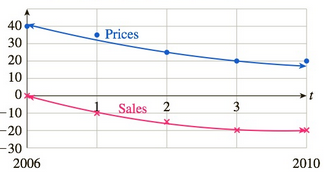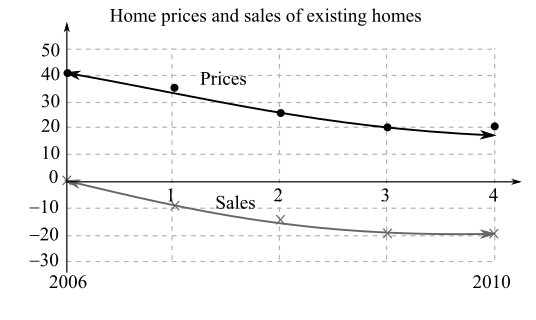Chapter 11.4, Problem 89EFinite Mathematics and Applied Cal...

7th Edition
Stefan Waner + 1 other
ISBN: 9781337274203

Solutions

Chapter
SectionFinite Mathematics and Applied Cal...

7th Edition
Stefan Waner + 1 other
ISBN: 9781337274203
Textbook Problem

Existing Home Sales The following graph shows the approximate value of home prices and existing home sales in 2006–2010 as a percentage change from 2003, together with quadratic approximations:Home prices and sales of existing homesThe quadratic approximations are given byHome prices: P ( t ) = t 2 − 10 t + 41      ( 0 ≤ t ≤ 4 ) Existing home sales: S ( t ) = 1.5 t 2 − 11 t      ( 0 ≤ t ≤ 4 ) , where t is time in years since the start of 2006. Use the chain rule to estimate d S d P | t = 2 . What does the answer tell you about home sales and prices? [HINT: See Quick Examples 6 and 7.]

To determine

To calculate: The derivative dSdP|t=2 using chain rule and also state what the answer conveys about home sales and prices if it is given that the approximate value of home prices and existing home sales in 20062010 as a percentage change from 2003, is given by,

Home prices: P(t)=t210t+41 such that (0t4).

Existing home prices: S(t)=1.5t211t such that (0t4).

The graph showing the approximations is given below:Explanation

Given Information:

The approximate value of home prices and existing home sales in 20062010 as a percentage change from 2003, is given by,

Home prices: P(t)=t210t+41 such that (0t4)

Existing home prices: S(t)=1.5t211t such that (0t4)

Where, t is time in years since the start of 2006.

The graph showing the approximations is shown below:

Formula used:

Derivative of function x and y using chain rule in differential notation is,

dydx=dydududx

Where, y is the differentiable function of u and u is the differentiable function of x.

Calculation:

Consider the function,

P(t)=t210t+41

Derivative of the function as shown below,

P(t)=ddt(t210t+41)=2t10

Consider the function,

S(t)=1

Still sussing out bartleby?

Check out a sample textbook solution.

See a sample solution

The Solution to Your Study Problems

Bartleby provides explanations to thousands of textbook problems written by our experts, many with advanced degrees!

Get Started

A sample with a mean of M = 8 has X = 56. How many scores are in the sample?

Essentials of Statistics for The Behavioral Sciences (MindTap Course List)

Evaluate the integral: 04x2x2ydy

Calculus: Early Transcendental Functions (MindTap Course List)

One of the vector forms of Green’s Theorem says that a) b) c) d)

Study Guide for Stewart's Multivariable Calculus, 8th

The interval of convergence of is: [−1, 1] [−1, 1) (−1, 1] (−1, 1)

Study Guide for Stewart's Single Variable Calculus: Early Transcendentals, 8th